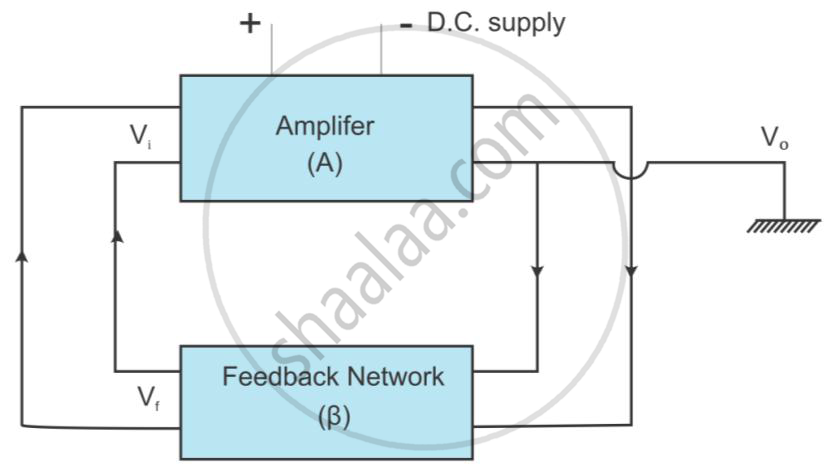# Explain the Elementary Idea of an Oscillator with the Help of Block Diagram - Physics

Explain the elementary idea of an oscillator with the help of block diagram

#### Solution

Oscillator:
An oscillator is an electronic device which generates an AC signal from a DC source. Oscillators are used in radio/TV receiver sets, radio/TV transmitters, RADAR, smartphones and microwave ovens. No input is applied to the oscillator, yet an output is obtained from it.

An oscillator requires an amplifier and a feedback network with frequency-determining components. When a part of the output of an amplifier is coupled to the input of the amplifier, it is called feedback. When the feedback sample is out of phase with the input, it is called negative feedback. When the feedback sample is in phase with the input, it is called positive feedback. For an oscillator, a positive feedback is required. The block diagram of an oscillator is shown below.The voltage gain of a complete system is given by

A_r=A/(1-Abeta)

where Af is the voltage gain with feedback, A is voltage gain without feedback and β is the feedback factor.

If for some frequency Aβ= 1, then the system gain becomes ∞ and the circuit begins to oscillate at that frequency. This condition (Aβ = 1) is called the Barkhausen criterion for sustained oscillations.
The frequency of oscillations depends on the LC or RC combinations used in a feedback network. When the power supply connected to the oscillator is turned ON, electrical noise of a wide range of frequencies is generated in the circuit, but the condition Aβ = 1 is satisfied only for a particular frequency and the oscillator oscillates at that frequency.

Concept: Oscillators
Is there an error in this question or solution?
2015-2016 (March)

Share